Courses

# Theory of Machines - 1

## 20 Questions MCQ Test Mock Test Series for SSC JE Mechanical Engineering | Theory of Machines - 1

Description
This mock test of Theory of Machines - 1 for SSC helps you for every SSC entrance exam. This contains 20 Multiple Choice Questions for SSC Theory of Machines - 1 (mcq) to study with solutions a complete question bank. The solved questions answers in this Theory of Machines - 1 quiz give you a good mix of easy questions and tough questions. SSC students definitely take this Theory of Machines - 1 exercise for a better result in the exam. You can find other Theory of Machines - 1 extra questions, long questions & short questions for SSC on EduRev as well by searching above.
QUESTION: 1

### Coriolis acceleration component of a slider moving at 75π mm/s on a link rotating at 60 rpm will be

Solution:

When a point on one link is sliding along another rotating link, then the Coriolis component of the acceleration is to be calculated.

ac=2ωV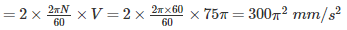QUESTION: 2

### In gears, interference takes place when _____.

Solution:

Due to interference, the tip of one of the gear of the pair will tend to dig into portions of the flank of the tooth of the other member of the pair.

QUESTION: 3

### Critical or whirling speed is the speed at which the shaft tends to vibrate violently in ________.

Solution:

Whirling speed or Critical speed of a shaft is defined as the speed at which a rotating shaft will tend to vibrate violently in the transverse direction if the shaft rotates in horizontal direction. In other words, the whirling or critical speed is the speed at which resonance occurs.

QUESTION: 4

A very soft whisper may have noise level of

Solution:

Some examples of different sound intensities as expressed in dB:

• 180 dB: Rocket at take-off
• 140 dB: Jet engine at take-off
• 120 dB: Rock band
• 110 dB: Loud thunder
• 90 dB: City traffic
• 60 dB: Ordinary conversation
• 30 dB: Soft whisper
• 0 dB: Softest sound a person can hear
QUESTION: 5

Which of the following statement is TRUE about the contact ratio?

Solution:

The ratio of the length of arc of contact to the circular pitch is known as contact ratio i.e. number of pairs of teeth in contact.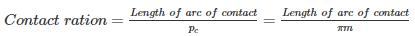QUESTION: 6

A pair of spur gears consists of a 20 teeth pinion meshing with a 120 teeth gear. The module is 4 mm. Calculate the centre distance (in mm).

Solution: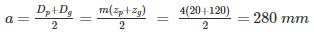QUESTION: 7

When one of the links of a kinematic chain is fixed, then the chain is called _______.

Solution:

When one of the links of a kinematic chain is fixed, the chain is known as mechanism. It may be used for transmitting or transforming motion. A mechanism with four links is known as simple mechanism, and the mechanism with more than four links is known as compound mechanism. Simplest possible mechanism is four bar chains consisting of four links, each of them forms a turning pair.

QUESTION: 8

A cam in which the follower reciprocates or oscillates in a plane parallel to the axis of the cam is known as

Solution:

A cylindrical cam or barrel cam is a cam in which the follower rides on the surface of a cylinder. In the most common type, the follower rides in a groove cut into the surface of a cylinder. These cams are principally used to convert rotational motion to linear motion parallel to the rotational axis of the cylinder.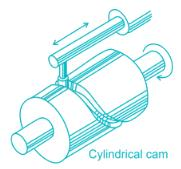QUESTION: 9

Governor is stable, when the:

Solution:

A governor is said to be stable when for every speed within the working range there is a definite configuration i.e. there is only one radius of rotation of the governor balls at which the governor is in equilibrium. For a stable governor, if the equilibrium speed increases, the radius of governor balls must also increase. A governor is said to be unstable, if the radius of rotation decreases as the speed increases.

QUESTION: 10

A ball and socket joint is example of _______ pair.

Solution:

When the two elements of a pair are connected in such a way that one element (with spherical shape) turns or swivels about the other fixed element, the pair formed is called a spherical pair. The ball and socket joint, attachment of a car mirror, pen stand etc., are the examples of a spherical pair.

QUESTION: 11

What will be the vertical height (m) of a watt governor, if the speed of rotation is 80 rpm?

Solution:

Height of the governor: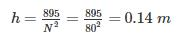QUESTION: 12

For a Hartnell governor, the loads on the spring at the lowest and highest equilibrium speeds are 1150 N and 85 N, respectively. If the lift of the governor is 1.5 cm, then spring stiffness would be

Solution:

let S be the stiffness of the spring, h be the lift of governor, Fand Fare the force exerted on sleeve at lowest and highest equilibrium speed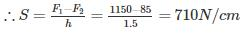QUESTION: 13

What is the radial distance of a tooth from the pitch circle to the top of the tooth known as?

Solution:

Addendum: It is the radial distance of a tooth from the pitch circle to the top of the tooth.

Dedendum: It is the radial distance of a tooth from the pitch circle to the bottom of the tooth.

Pitch circle: It is an imaginary circle which by pure rolling action, would give the same motion as the actual gear.

Pitch circle diameter: It is the diameter of the pitch circle. The size of the gear is usually specified by the pitch circle diameter. It is also called as pitch diameter.

Module: It is the ratio of the pitch circle diameter in millimetres to the number of teeth.

Module, m = D / T

QUESTION: 14

Elliptical gear train used in differential gear of automobile helps in:

Solution:

When a vehicle takes a turn, its other rear wheel covers a greater distance than inner rear wheel. Hence differential gears are used so that both the rear wheel can rotate at different speed. This is achieved with the help of elliptical gear train.

QUESTION: 15

The mass of flywheel of a steam engine is 3250 kg with the radius of gyration of 1 m. The starting torque of the engine is 4500 N - m. What is the angular acceleration (rad/s2) of the flywheel?

Solution:

Mass moment of inertia of the flywheel:

I = mk2 = 3250 × 12 = 3250 kg-m2

Starting torque of the engine: T = I.α

Angular acceleration of the flywheel:

α = T/I = 4500/3250 = 18/13 = 1.38 rad/s2

QUESTION: 16

________ gear train is used to connect minute hand to hour hand, in a clock mechanism.

Solution:

When the axes of the first gear and the last gear are co-axial, then the gear train is known as reverted gear train. The reverted gear trains are used in automotive transmissions, lathe back gears, industrial speed reducers, and in clocks (where the minute and hour hand shafts are co-axial).

The epicyclic gear trains are useful for transmitting high velocity ratios with gears of moderate size in a comparatively lesser space. The epicyclic gear trains are used in the back gear of lathe, differential gears of the automobiles, hoists, pulley blocks, wrist watches etc.

QUESTION: 17

A cantilever beam of cross section area ‘A’, moment of Inertia I and length ‘L’ is having natural frequency ω1. If the beam is accidentally broken into two halves, the natural frequency of the remaining cantilever beam ω2will be such that

Solution: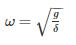For cantilever beam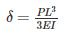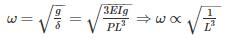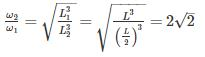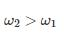QUESTION: 18

In case of cam, the maximum value of the pressure angle is kept as-

Solution:

Pressure Angle is the measure of steepness of the cam profile. The angle between the direction of the follower movement and the normal to the pitch curve at any point is called pressure angle. Pressure angle varies from maximum to minimum during complete rotation.

The pressure should not exceed 30 in case of cams with translating followers (and angle should be less than 45 for low speed cam mechanisms with oscillating followers).

QUESTION: 19

________ mechanism produces mathematically an exact straight line motion.

Solution:

Exact Straight-Line Motion Mechanisms Made up of Turning Pairs: Peaucellier mechanism, Hart’s mechanism

Exact Straight-Line Motion Consisting of One Sliding Pair: Scott Russell’s Mechanism

Approximate Straight-Line Motion Mechanisms: Watt’s mechanism, Modified Scott-Russel mechanism, Grasshopper mechanism, Roberts mechanism

QUESTION: 20

Pick up the wrong statement. A flywheel

Solution:

A flywheel controls the speed variation caused by the engine whereas governor maintains a constant speed during load variation on the engine.